# Scikit-learn聚类方法代码批注及相关练习

### 一、代码批注

``````import numpy as np
from sklearn.cluster import DBSCAN
from sklearn import metrics
from sklearn.datasets import make_blobs
from sklearn.preprocessing import StandardScaler

# make_blobs:为聚类产生数据集及其相应的标签；n_samples：样本点个数；centers：类别数；cluster_std：每个类别的方差；random_state：随机种子
# 这里centers里的三个二维坐标，其实代表了聚类的三个中心
centers = [[1, 1], [-1, -1], [1, -1]]
X, labels_true = make_blobs(n_samples=750, centers=centers, cluster_std=0.4, random_state=0)

# 标准化
X = StandardScaler().fit_transform(X)

# 预估器，并得出模型（eps：数据点的邻域半径；min_samples：某个数据点的邻域内最少有的数据点个数）
db = DBSCAN(eps=0.2, min_samples=7).fit(X)

# 生成n_samples个False
# 预测出的结果（有结果的为True，为噪音的是False）
# 获得预测结果
labels = db.labels_
# 获得预测的聚类数，忽略掉噪音
n_clusters_ = len(set(labels)) - (1 if -1 in labels else 0)
# 获得噪音数
n_noise_ = list(labels).count(-1)

# 预测出的集群数，对应图中5中颜色
print('Estimated number of clusters: %d' % n_clusters_)
# 预测出噪音点的数量，对应图中的黑点
print('Estimated number of noise points: %d' % n_noise_)
# 同质性：簇的纯洁程度—对比分类问题的精度
print("Homogeneity: %0.3f" % metrics.homogeneity_score(labels_true, labels))
# 完整性：簇的完整性—对比分类问题的召回率
print("Completeness: %0.3f" % metrics.completeness_score(labels_true, labels))
# v测度：用来评估同一个数据集上两个独立赋值的一致性
print("V-measure: %0.3f" % metrics.v_measure_score(labels_true, labels))
# 调节的兰德系数（ARI）：衡量两个数据分布的吻合程度
# 调整互信息（AMI）：衡量两个数据分布的吻合程度
# 轮廓系数：将某个对象与自己的簇的相似程度和与其他簇的相似程度进行比较（肘方法）
print("Silhouette Coefficient: %0.3f" % metrics.silhouette_score(X, labels))

import matplotlib.pyplot as plt

unique_labels = set(labels)
# 给每个label赋个颜色
colors = [plt.cm.Spectral(each) for each in np.linspace(0, 1, len(unique_labels))]
for k, col in zip(unique_labels, colors):
# 给噪音为黑色
if k == -1:
col = [0, 0, 0, 1]

# 开始绘制，获得该种类的点
plt.plot(xy[:, 0], xy[:, 1], 'o', markerfacecolor=tuple(col), markeredgecolor='k', markersize=14)
# 获得噪音点，注意：db.core_sample_indices_没出现的的不一定就是噪音点（小圆圈）
plt.plot(xy[:, 0], xy[:, 1], 'o', markerfacecolor=tuple(col), markeredgecolor='k', markersize=6)

plt.title('Estimated number of clusters: %d' % n_clusters_)
plt.show()
``````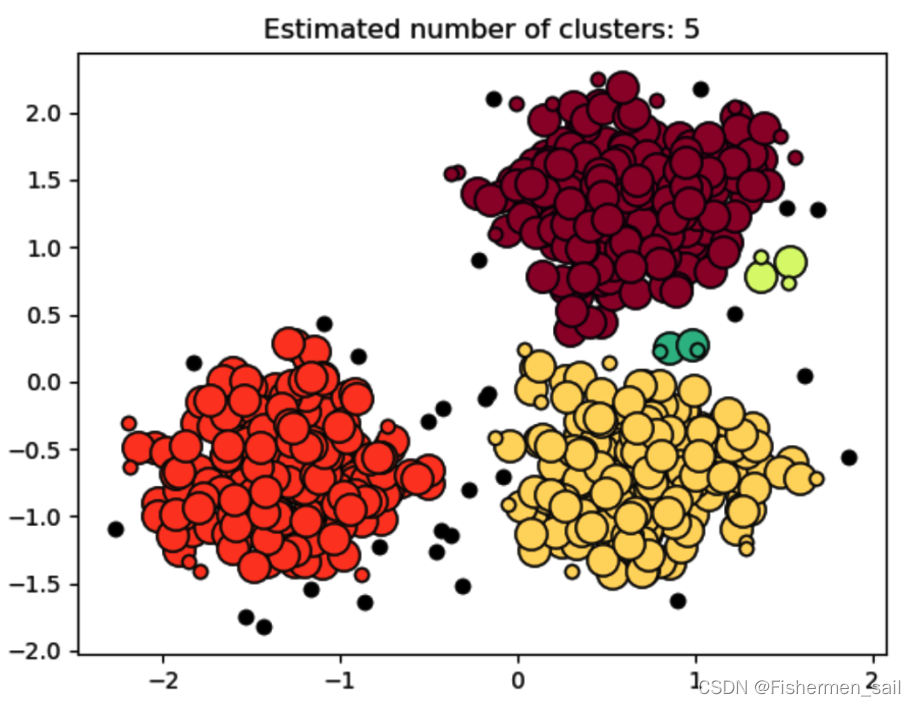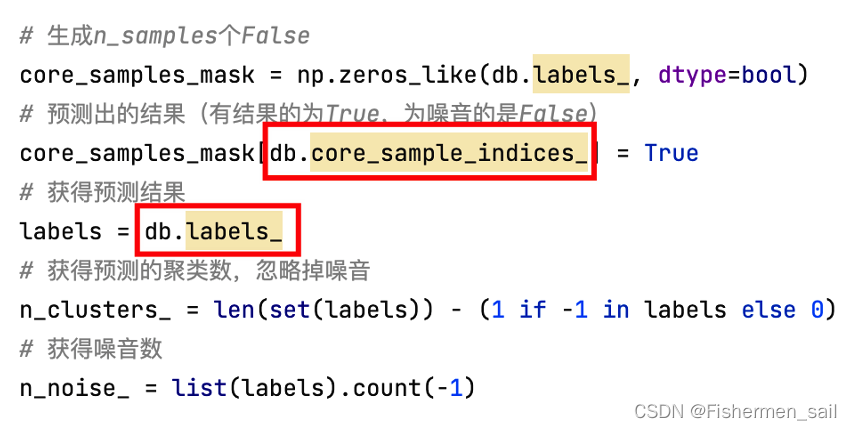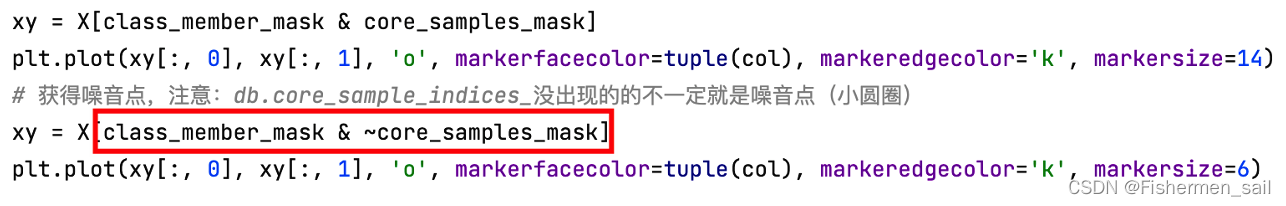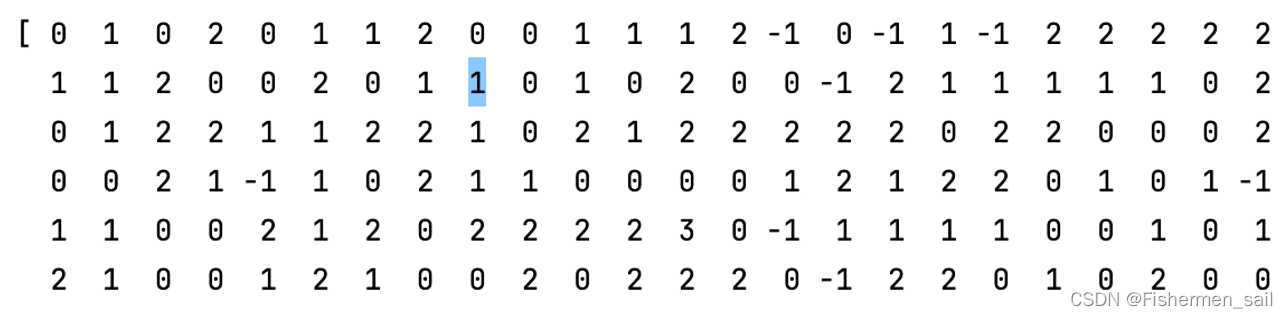### 二、DBSCAN的使用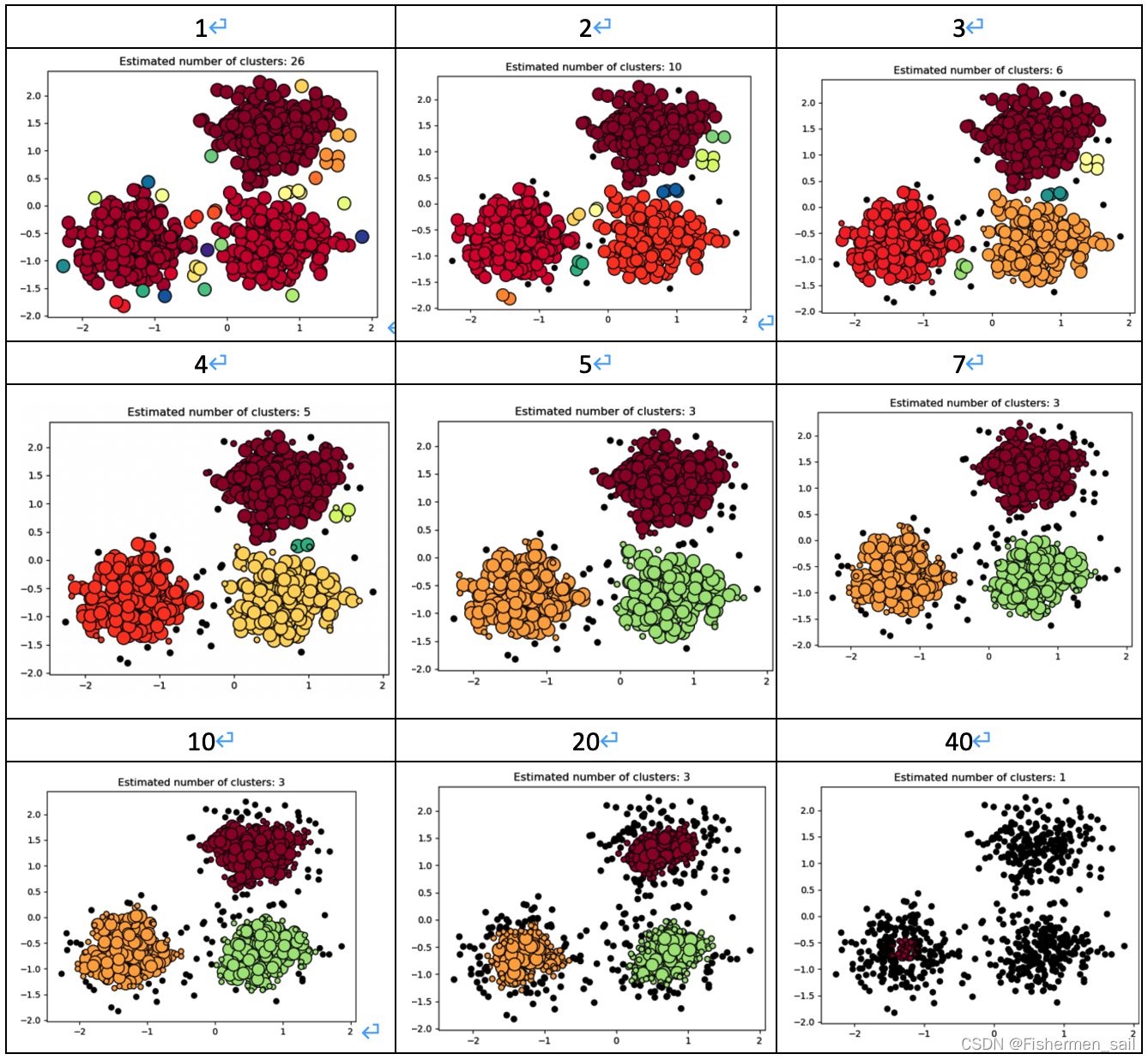eps表示数据点的邻域半径，如果某个数据点的邻域内至少有min_sample个数据点，则将该数据点看作为核心点。如果某个核心点的邻域内有其他核心点，则将它们看作属于同一个簇。如果min_sampLes设置地太大，那么意味着更少的点会成为核心点，而更多的点将被标记为噪声。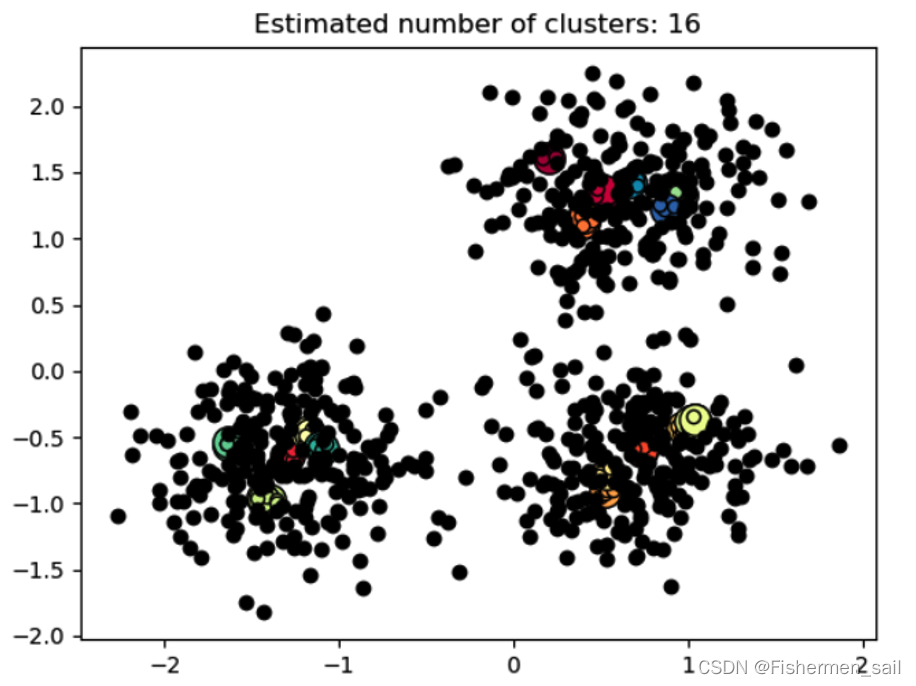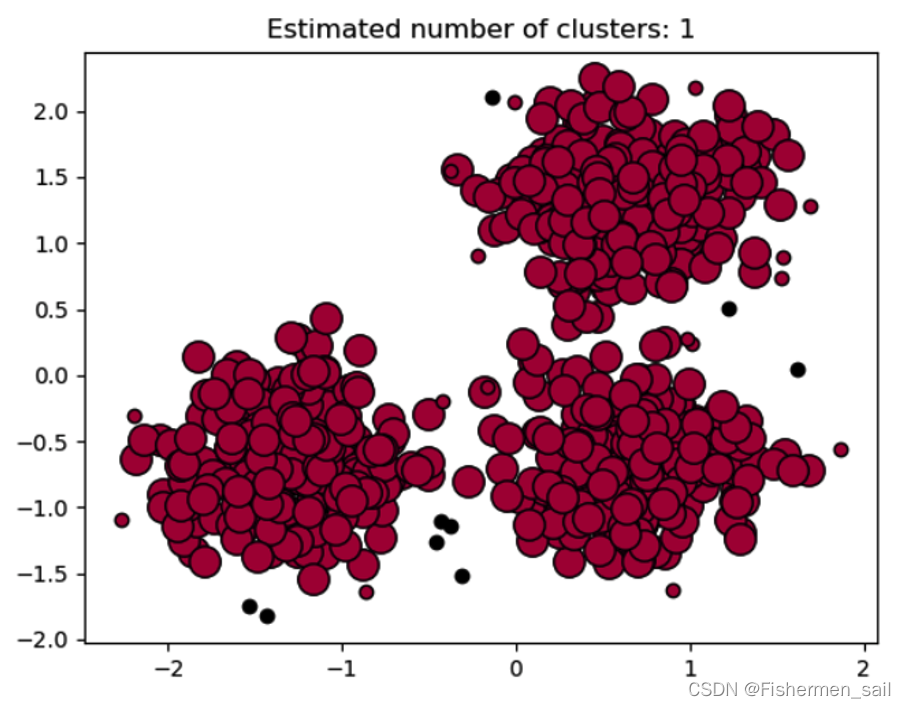### 三、KMeans的使用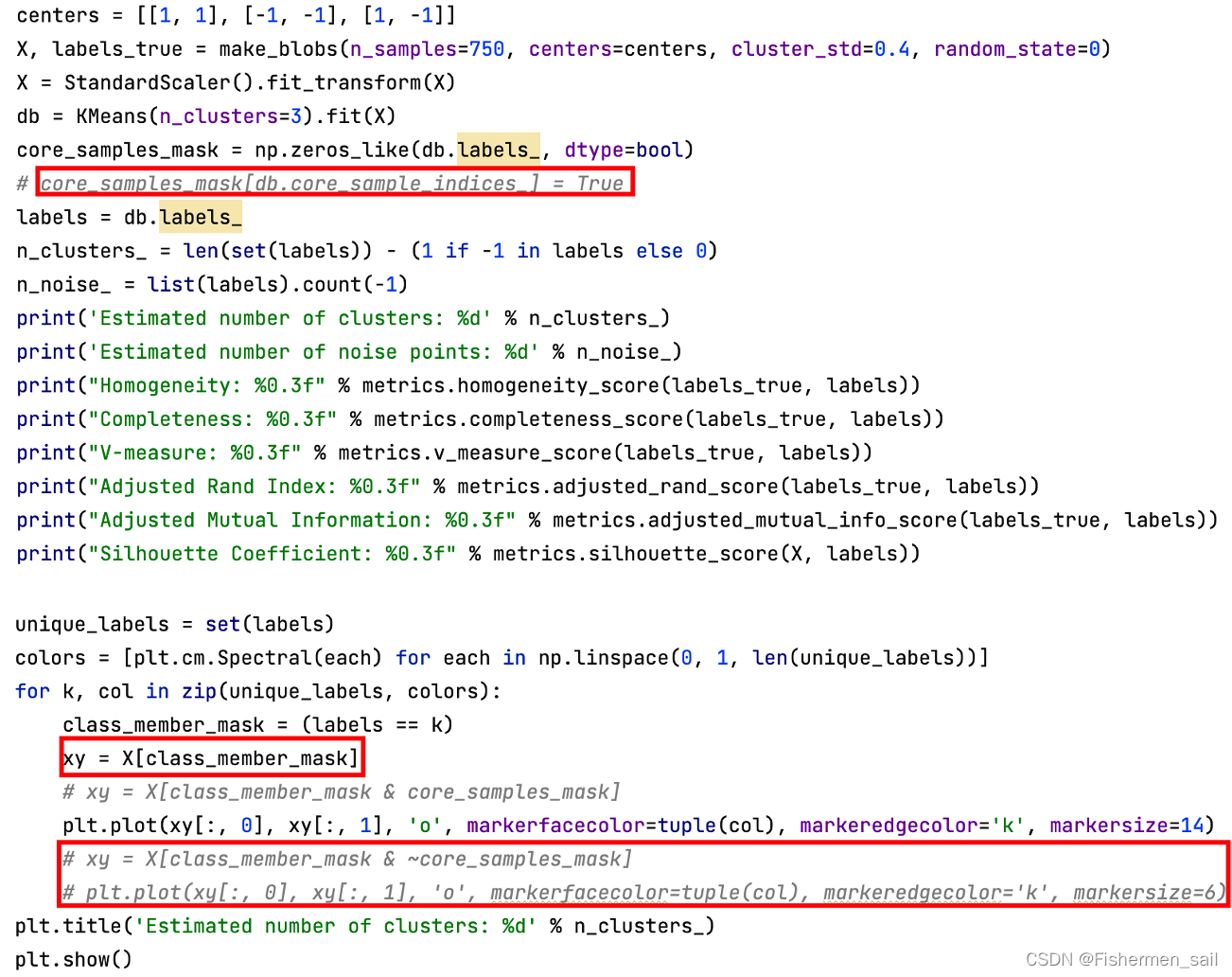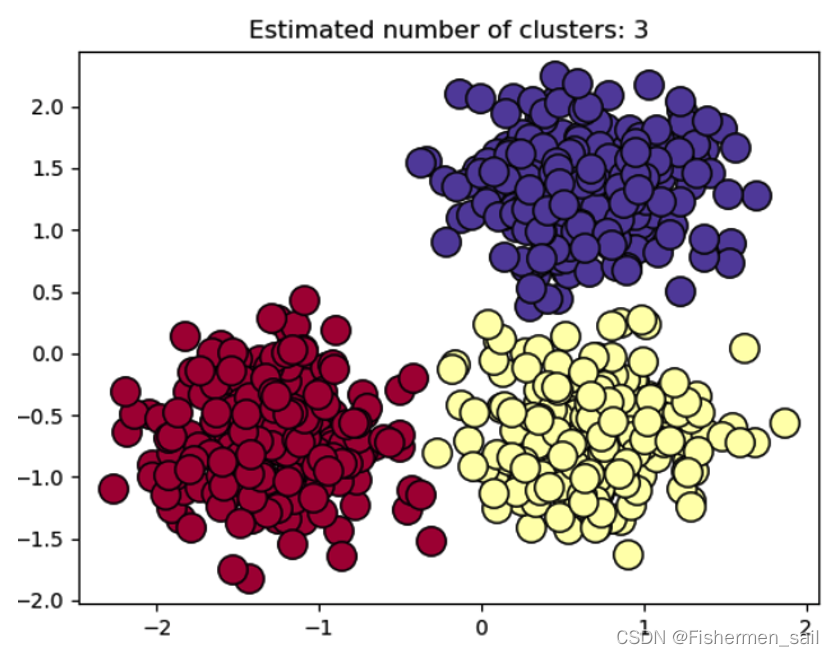KMeans算法是根据给定的n个数据对象的数据集，构建n个划分聚类的方法，每个划分聚类即为一个簇。该方法将数据划分为n个簇，每个簇至少有一个数据对象，每个数据对象必须属于而且只能属于一个簇。同时要满足同一簇中的数据对象相似度高，不同簇中的数据对象相似度较小。聚类相似度是利用各簇中对象的均值来进行计算的。

KMeans 算法的处理流程如下，首先，随机地选择k个数据对象，每个数据对象代表一个簇中心，即选择k个初始中心；对剩余的每个对象，根据其与各簇中心的相似度（距离），将它赋给与其最相似的簇中心对应的簇；然后重新计算每个簇中所有对象的平均值，作为新的簇中心。不断重复以上这个过程，直到准则函数收敛，也就是簇中心不发生明显的变化。通常采用均方差作为准则函数，即最小化每个点到最近簇中心的距离的平方和。新的簇中心计算方法是计算该簇中所有对象的平均值，也就是分别对所有对象的各个维度的值求平均值，从而得到簇的中心点。

KMeans DBSCAN